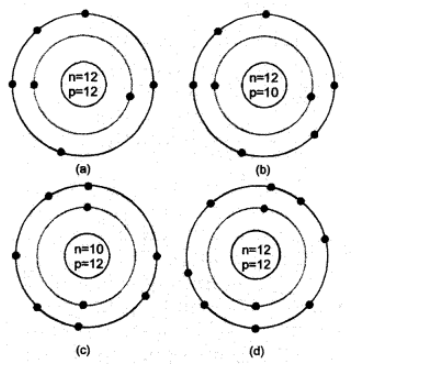# identify the mg2+ ion from the given`
Question:

Identify the $\mathrm{Mg}^{2+}$ ion from the given figure where $\mathrm{n}$ and $\mathrm{p}$ represent the number of neutrons and protons respectivelySolution:

(d) Since the ion has 10 electrons, it is $\mathrm{Mg}^{2+}$ ion.